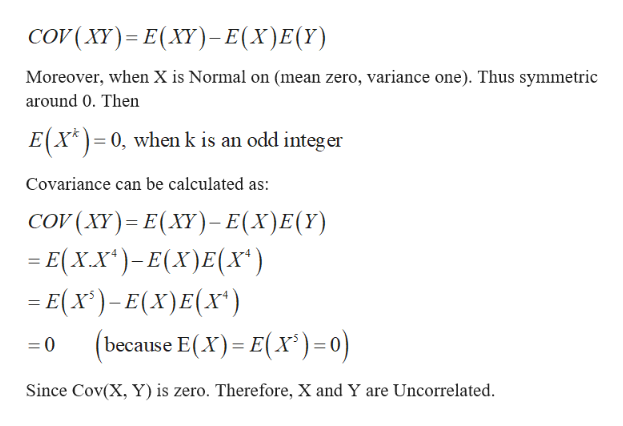# LetX have a standard normal distribution (mean zero, variance one) and letY=X 4. Show that X and Y are uncorrelated. Are X and Y independent?

Question
48 views

LetX have a standard normal distribution (mean zero, variance one) and letY=X 4. Show that X and Y are uncorrelated. Are X and Y independent?

check_circle

Step 1

Two R.V’s X, Y are uncorrelated if their co...help_outlineImage Transcriptionclosecov ( XY) - Ε(ΧΥ) - Ε(Χ) E() Moreover, when X is Normal on (mean zero, variance one). Thus symmetric around 0. Then E(X* )= 0, when k is an odd integer Covariance can be calculated as: COV (XY)= E(XY)- E(X)E(Y) = E(X.X*)-E(X)E(Xx*) = E(x*)– E(X)E(x*) because E(X)= E(X³)=0) Since Cov(X, Y) is zero. Therefore, X and Y are Uncorrelated. fullscreen

### Want to see the full answer?

See Solution

#### Want to see this answer and more?

Solutions are written by subject experts who are available 24/7. Questions are typically answered within 1 hour.*

See Solution
*Response times may vary by subject and question.
Tagged in

### Other